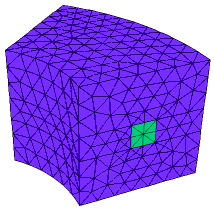﻿ Sample Problems > Usage > Import_Export > 3d_mesh_import

# 3d_mesh_import

Navigation:  Sample Problems > Usage > Import_Export >

# 3d_mesh_import{ 3D_MESH_IMPORT.PDE

This example shows the use of the TRANSFERMESH command to import a

3D Mesh.  The mesh file is created by running 3D_MESH_EXPORT.PDE.

Note that the domain structure must exactly match that of the exporting problem.

Periodicity condtions must also be the same, except that periodic and antiperiodic

may be exchanged.

(The framework of this problem is 3D_ANTIPERIODIC.PDE.)

}

 title '3D MESH IMPORT TEST'   coordinates cartesian3   variables    u   definitions    k = 1   { angular size of the repeated segment: }    an = pi/4           { sine and cosine needed in transformation }    crot = cos(an)      srot = sin(an)    H = 0    xc = 1.5    yc = 0.2    rc = 0.1     transfermesh("3d_mesh_export_output/mesh3.xfr")       ! << read the mesh file   equations    U: div(K*grad(u)) + H = 0   extrusion z=0,0.4,0.6,1   boundaries   Region 1start(1,0) line to (2,0)

value(u) = 0 arc(center=0,0) to (2*crot,2*srot)

antiperiodic(x*crot+y*srot, -x*srot+y*crot)

line to (crot,srot)

natural(u)=x-2.4*y       ! BC changed from exporting problem

arc(center= 0,0) to close

Limited Region 2

layer 2 H = 1

start(xc-rc,0) line to (xc+rc,0) to (xc+rc,rc) to (xc-rc,rc) to close

Limited Region 3

layer 2 H = -1

start((xc-rc)*crot,(xc-rc)*srot)

line to ((xc+rc)*crot,(xc+rc)*srot)

to ((xc+rc)*crot+rc*srot,(xc+rc)*srot-rc*crot)

to ((xc-rc)*crot+rc*srot,(xc-rc)*srot-rc*crot) to close

plots

contour(u) on z=0.5 paint

grid(x,y,z)

end